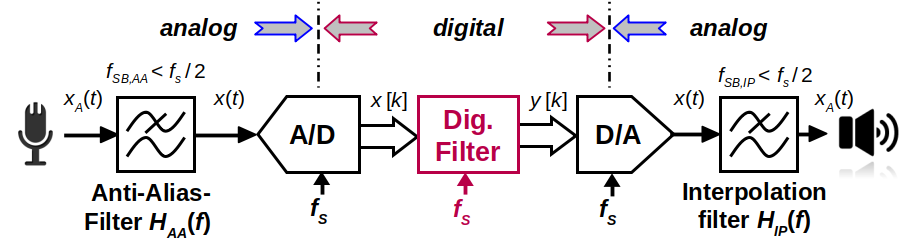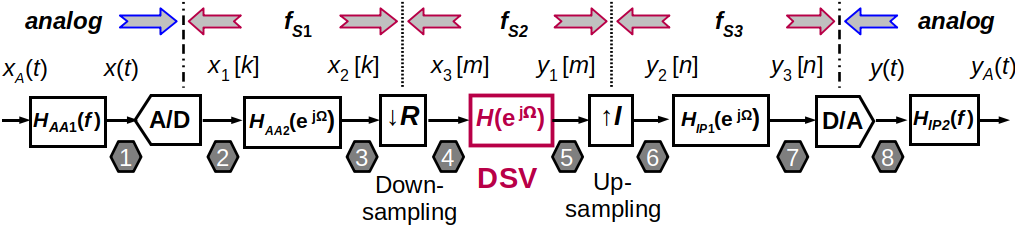# Input Specs¶

Fig. 2 shows a typical view of the Specs tab where you can specify the kind of filter to be designed and its specifications in the frequency domain:

• Response type (low pass, band pass, …)
• Filter type (IIR for a recursive filter with infinite impulse response or FIR for a non-recursive filter with finite impulse response)
• Filter class (elliptic, …) allowing you to select the filter design algorithm

Not all combinations of design algorithms and response types are available - you won’t be offered unavailable combinations and some fields may be greyed out.

## Order¶

The order of the filter, i.e. the number of poles / zeros / delays is either specified manually or the minimum order can be estimated for many filter algorithms to fulfill a set of given specifications.

## Frequency Unit¶

In DSP, specifications and frequencies are expressed in different ways:

$F = \frac{f}{f_S} \textrm{ or }\Omega = \frac{2\pi f}{f_S} = 2\pi F$

In pyfda, you can enter parameters as absolute frequency $${{f}}$$, as normalized frequency $${{F}}$$ w.r.t. to the Sampling Frequency $${f_S}$$ or to the Nyquist Frequency $$f_{Ny} = f_S / 2$$ (Fig. 3):

## Amplitude Unit¶

Amplitude specification can be entered as V, dB or W; they are converted automatically. Conversion depends on the filter type (IIR vs. FIR) and whether pass or stop band are specified. For details see the conversion functions pyfda.libs.pyfda_lib.unit2lin() and pyfda.libs.pyfda_lib.lin2unit().

## Background Info¶

### Sampling Frequency¶

One of the most important parameters in a digital signal processing system is the sampling frequency $${\pmb{f_S}}$$, defining the clock frequency with which the registers (flip-flops) in the system are updated. In a simple DSP system, the clock frequency of ADC, digital filter and DAC might be identical:Fig. 4 A simple signal processing system

Sometimes it makes sense to change the sampling frequency in the processing system e.g. to reduce the sampling rate of an oversampling ADC or to increase the clocking frequency of an DAC to ease and improve reconstruction of the analog signal.Fig. 5 A signal processing system with multiple sampling frequencies

### Aliasing and Nyquist Frequency¶

When the sampling frequency is too low, significant information is lost in the process and the signal cannot be reconstructed without errors (forth image in Fig. 6) [Smith99]. This effect is called aliasing.Fig. 6 Sampling with $$f_S = 1000$$ Hz of sinusoids with 4 different frequencies

When sampling with $$f_S$$, the maximum signal bandwidth $$B$$ that can represented and reconstructed without errors is given by $$B < f_S/2 = f_{Ny}$$. This is also called the Nyquist frequency or bandwidth $$f_{Ny}$$. Some filter design tools and algorithms normalize frequencies w.r.t. to $$f_{Ny}$$ instead of $$f_S$$.